8146.0 - Household Use of Information Technology, Australia, 2012-13
ARCHIVED ISSUE Released at 11:30 AM (CANBERRA TIME) 25/02/2014
 Page tools: .mffeedback,#pjs { display :none; } Enable Javascript to Print PagesPrint All TECHNICAL NOTE DATA QUALITY INTRODUCTION 1 When interpreting the results of a survey it is important to take into account factors that may affect the reliability of the estimates. Estimates in this publication are subject to both non-sampling and sampling errors. NON-SAMPLING ERROR 2 Non-sampling errors may arise as a result of errors in the reporting, recording or processing of the data and can occur even if there is a complete enumeration of the population. These errors can be introduced through inadequacies in the questionnaire, treatment of non-response, inaccurate reporting by respondents, errors in the application of survey procedures, incorrect recording of answers and errors in data capture and processing. 3 The extent to which non-sampling error affects the results of the survey is difficult to measure. Every effort is made to reduce non-sampling error by careful design and testing of the questionnaire, training and supervision of interviewers and extensive quality control procedures at all stages of data processing. SAMPLING ERROR 4 Since the estimates in this publication are based on information obtained from a sample, they are subject to sampling variability. That is, they may differ from those estimates that would have been produced if all dwellings had been included in the survey. One measure of the likely difference is given by the standard error (SE), which indicates the extent to which an estimate might have varied by chance because only a sample of dwellings (or occupants) was included. There are about two chances in three (67%) that a sample estimate will differ by less than one SE from the number that would have been obtained if all dwellings had been included, and about 19 chances in 20 (95%) that the difference will be less than two SEs. 5 Another measure of the likely difference is the relative standard error (RSE), which is obtained by expressing the SE as a percentage of the estimate: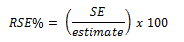6 RSEs for HUIT statistics have been calculated using the Jackknife method of variance estimation. This involves the calculation of 30 'replicate' estimates based on 30 different subsamples of the obtained sample. The variability of estimates obtained from these subsamples is used to estimate the sample variability surrounding the estimates. 7 In this publication, sampling variability is indicated through use of annotations which have been produced using RSEs. RSEs for all data included in this release are available in Data cubes 3 and 4 in the Downloads section. 8 Only estimates (numbers and proportions) with RSEs less than 25% are considered sufficiently reliable for most purposes. Estimates with RSEs between 25% and 50% have been included and are annotated to indicate they are subject to high sample variability and should be used with caution. Estimates with RSEs greater than 50% are annotated to indicate that they are considered too unreliable for general use. CALCULATION OF STANDARD ERRORS 9 SEs can be calculated using the estimates (counts or means) and the corresponding RSEs: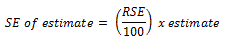10 For example, Table 2 of Data cube 1 shows that the estimated number of households with broadband internet access in 2012–13 is 6,801,000. In the corresponding RSE table, the RSE for this estimate is shown to be 1%. Applying the above formula, the SE is: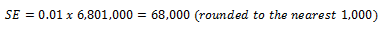11 Therefore there are about two chances in three that the value that would have been produced if all dwellings had been included in the survey will fall within the range (6,733,000 to 6,869,000) and about 19 chances in 20 that the value will fall within the range (6,665,000 to 6,937,000). PROPORTIONS AND PERCENTAGES 12 Proportions and percentages formed from the ratio of two estimates are also subject to sampling errors. The size of the error depends on the accuracy of both the numerator and the denominator. A formula to approximate the RSE of a proportion is given below. This formula is only valid when x is a subset of y.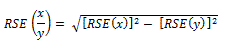13 For example, the estimated number of internet users in Tasmania was 325,000 persons (with an RSE of 1%) and the estimated number of internet users in Tasmania who used the internet to purchase or order goods or services was 257,000 (with an RSE of 2%). Therefore the proportion of Tasmanian internet users who used the internet to purchase or order goods or services and access out of all Tasmanian internet users is 79% (as seen in Table 4 of Data cube 2). 14 Applying the above formula, the RSE of this proportion is calculated as: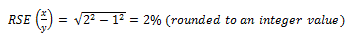15 Calculation of the SE for the proportion of Tasmanian internet users who used the internet to purchase or order goods or services and access is as follows: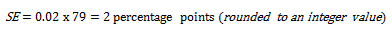16 Therefore there are about two chances in three that the proportion of internet users in Tasmania who used the internet to purchase or order goods or services will fall within the range (77% and 81%) and 19 chances in 20 that the proportion is within the range (75% to 83%). DIFFERENCES 17 Published estimates may also be used to calculate the difference between two survey estimates (of numbers or percentages). Such an estimate is subject to sampling error. The sampling error of the difference between two estimates depends on their SEs and the relationship (correlation) between them. An approximate SE of the difference between two estimates (x-y) may be calculated by the following formula: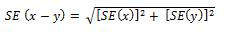18 While this formula will only be exact for differences between separate and uncorrelated characteristics or subpopulations, it provides a good approximation for all differences likely to be of interest in this publication. SIGNIFICANCE TESTING 19 A statistical significance test for any comparisons between estimates can be performed to determine whether it is likely that there is a difference between the corresponding population characteristics. The standard error of the difference between two corresponding estimates (x and y) can be calculated using the formula in the Differences section above. This standard error is then used to calculate the following test statistic: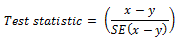20 If the value of this test statistic is greater than 1.96 then there is evidence, with a 95% level of confidence, of a statistically significant difference in the two populations with respect to that characteristic. Otherwise, it cannot be stated with confidence that there is a real difference between the populations with respect to that characteristic. Document Selection These documents will be presented in a new window.